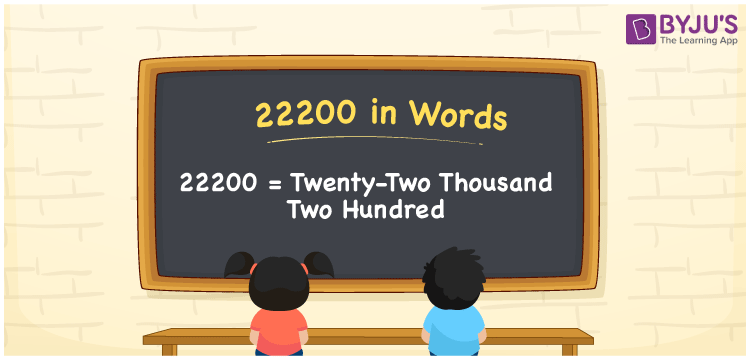# 22200 in Words

22200 in words is written as Twenty-two thousand two hundred. In both the International System of Numerals and the Indian System of Numerals, 22200 is written as Twenty-two thousand two hundred. The number 22200 is a Cardinal Number as it represents some quantity. For example, “that Smart-TV costs 22200 rupees”, 22200 represents the TV cost.

 22200 in Words Twenty-two thousand two hundred Twenty-two thousand two hundred in Number 22200

## 22200 in English Words

22200 in English words is read as “Twenty-two thousand two hundred.”## How to Write 22200 in Words?

To write 22200 in words, we shall use the place value chart. In the place value chart, put 2 in the ten thousands, thousands, and hundreds, and 0 in the tens and the ones, respectively. Let us make a place value chart to write the number 22200 in words.

 Ten Thousands Thousands Hundreds Tens Ones 2 2 2 0 0

Thus, we can write the expanded form as

2 × Ten Thousand + 2 × Thousand + 2 × Hundred + 0 × Ten + 0 × One

= 2 × 10000 + 2 × 1000 + 2 × 100 + 0 × 10 + 0 × 1

= 20000 + 2000 + 200 + 0 + 0

= 22200

= Twenty-two thousand two hundred.

22200 is a natural number, the successor of 22199 and the predecessor of 22201.

22200 in words – Twenty-two thousand two hundred

• Is 22200 an odd number? – No
• Is 22200 an even number? – Yes
• Is 22200 a perfect square number? – No
• Is 22200 a perfect cube number? – No
• Is 22200 a prime number? – No
• Is 22200 a composite number? – Yes

## Frequently Asked Questions on 22200 in Words

Q1

### How to write 22200 in words?

22200 in words is written as Twenty-two thousand two hundred.
Q2

### How to write 22200 in words in the International and Indian System of Numerals?

In both, the system of numerals, 22200 in words, is written as Twenty-two thousand two hundred.
Q3

### What is the successor and predecessor of 22200?

The successor of 22200 is 22201, and the predecessor of 22200 is 22199.